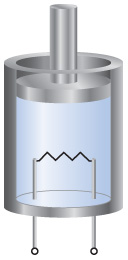# Problem: A gas is confined to a cylinder fitted with a piston and an electrical heater, as shown here:Suppose that current is supplied to the heater so that 100 J of energy is added. Consider two different situations. In case (1) the piston is allowed to move as the energy is added. In case (2) the piston is fixed so that it cannot move.In which case is E for the system (the gas in the cylinder) larger?

###### FREE Expert Solution

Internal Energy:

$\overline{){\mathbf{∆}}{\mathbf{E}}{\mathbf{=}}{\mathbf{q}}{\mathbf{+}}{\mathbf{w}}}$

• Case 1 → energy is added (+) to the system, w < 0 (-) since work is done by the system to surroundings (can expand):
• ΔE = 100 J + (-w)
89% (312 ratings)###### Problem Details

A gas is confined to a cylinder fitted with a piston and an electrical heater, as shown here:Suppose that current is supplied to the heater so that 100 J of energy is added. Consider two different situations. In case (1) the piston is allowed to move as the energy is added. In case (2) the piston is fixed so that it cannot move.

In which case is E for the system (the gas in the cylinder) larger?

Frequently Asked Questions

What scientific concept do you need to know in order to solve this problem?

Our tutors have indicated that to solve this problem you will need to apply the Internal Energy concept. You can view video lessons to learn Internal Energy. Or if you need more Internal Energy practice, you can also practice Internal Energy practice problems.